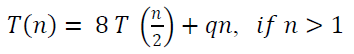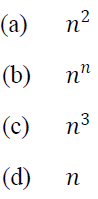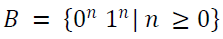1. The running time of an algorithm 𝑇(𝑛 ) where 𝑛 the input size is given by:= 𝑝 , if 𝑛 = 1
where, 𝑝 and 𝑞 are constants.

What is the complexity (order) of the algorithm?2. Consider the following statements regarding automata theory:
1. The pumping length must always be equal to the number of states in a machine
2. A non-regular expression can have a finite pumping length
3. In a regular language/expression, a string of pumping length can be repeated arbitrarily
3. The languagehas a pumping length

Which of the above statements is/are correct?
(a) 1, 2 and 4
(b) 1, 3 and 4
(c) 2 only
(d) 3 only

4. Consider the following machines regarding Finite automatas:
1. DFA
2. NFA
3. E - NFA
4. Any automaton

Which of the above are correct about the applicability of Arden’s Theorem?
(a) 1 and 4
(b) 1 and 2
(c) 2 and 3
(d) 3 and 4

5. In C programming, the qualifiers ‘signed’ and ‘unsigned’ apply to
1. Char
2. Float
3. Int
4. Double

(a) 1 and 4
(b) 2 and 3
(c) 1 and 3
(d) 2 and 4

6. When a compiler encounters a function parameter for a single-subscripted array of the form int a [ ], it converts the parameter to:
(a) Int a
(b) Int & a
(c) Int * a
(d) No conversion is required

7. The dominator node in DAG represents
(a) Any node of DAG which represents start of loop
(b) Last node of DAG
(c) Node with highest degree of DAG
(d) Isolated node of DAG

8. Which of the following is used for grouping of characters into tokens?
(a) Parser
(b) Code optimization
(c) Code generator
(d) Lexical analyzer

9. Consider the following contents of different registers:

Offset (displacement) = 5000 H
[AX]−1000 𝐻 , [BX]−2000𝐻 , [SI]−4000𝐻 , [DI]−3000𝐻
[BP]−5000 𝐻 , [SP]−6000𝐻 , [CS]−0000𝐻 , [DS]−2000𝐻
[SS]−3000 𝐻 , [IP]−7000𝐻 ,

What is the effective address of the data for the following instruction?

MOV  AX,  [BX ] [SI]

(a) 20000𝐻
(b) 25000𝐻
(c) 26000𝐻
(d) 30000𝐻